## GroveStreams Energy Cost Tutorial

This tutorial walks through:
This example demonstrates how to derive a monthly cost stream from an expression that converts amperes to kWh while applying price per kWh. There are several ways that kWh can be calculated from current. This example uses the monthly ampere average to estimate kWh. Contact your utility company to determine how your meter is calculating kWh to calculate more accurate costs.

The utility rate used in this example is a simple price per kilowatt hour rate. GroveStreams can calculate more complex time-of-use block rates. These types of rates are discussed at the end of the tutorial.

This tutorial assumes you have followed one of our Feed examples and have programmed your device to upload current samples. A live example of the tutorial can be found by creating a GroveStreams Sandbox organization. This tutorial is based on the sandbox smart plug which monitors a refrigerator's current every second. Create your own sandbox organization to investigate the smart plug component and its energy cost modeling in more detail without having to program your own device to upload a current stream.

### 1. Using a current (Amperes) stream to calculate monthly energy costs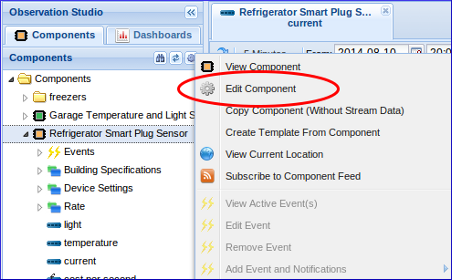Edit the component definition: Right click on your component and select Edit Component.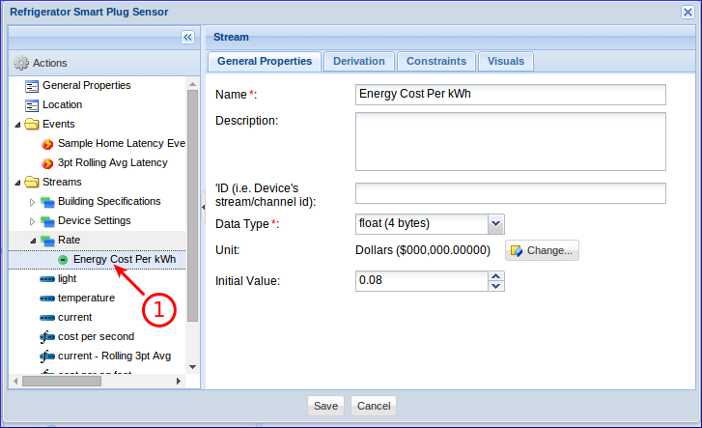Right click on the Streams folder and create a new Point Stream. Give the stream the same settings as above. This point stream will hold the price per kWh (0.08 in the example above) and is used in the derivation formula below. Change 0.08 to your actual price per kWh.

Tip: Create a separate component that only contains the energy price stream if there are many components using the same energy price. Use that stream in all of your energy derivation formulas. This prevents having to edit the price for many streams whenever it changes.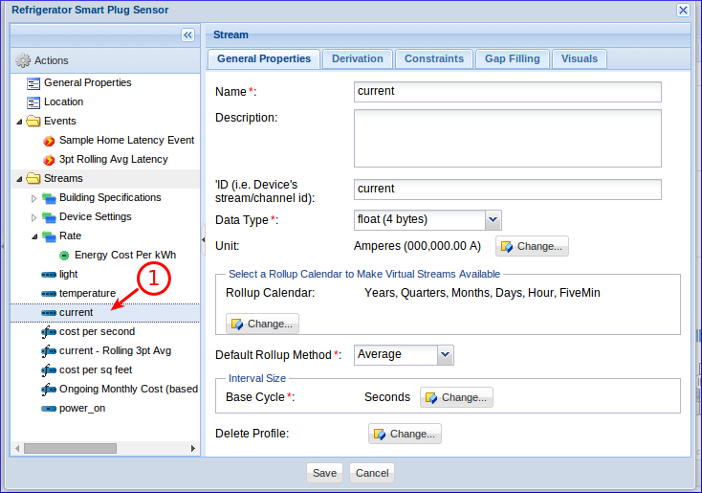Verify the current stream is an Interval Stream and has a Rollup Calendar assigned that includes the Month Cycle. The Base Cycle of the stream is set to One Second (it is recording current every second). You may select a different Base Cycle that matches your sample frequency.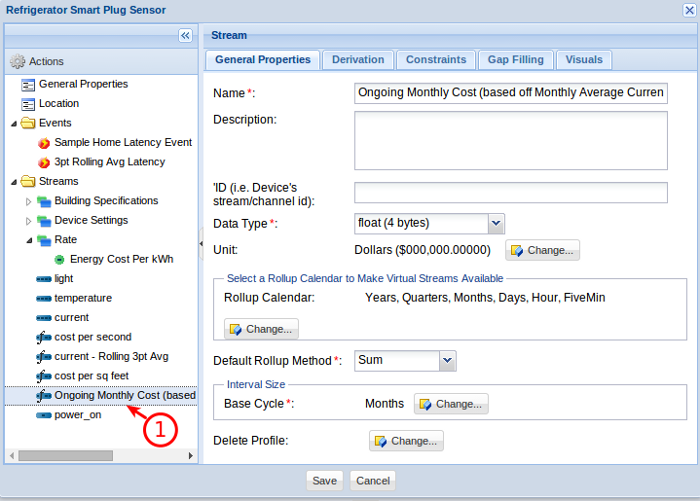Create the energy cost stream:
1. Right click on the Streams folder and select Add - Stream - Interval Stream. Fill in the fields with the settings above.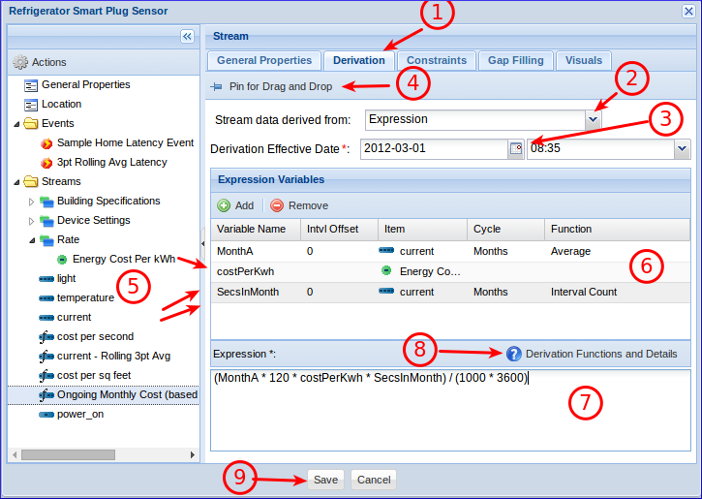Configure the cost stream to be derived:
1. Select the Derivation tab.
2. Set Stream data derived from to Expression.
3. Optionally set the Derivation effective Date to a date and time earlier than any existing current samples.
4. Toggle the Pin for Drag and Drop button. This will allow streams to be selected and dragged without changing the view.
5. Drag and drop the "Energy Cost Per kWh" stream onto the Expression Variables grid. Drag and drop the "current" stream twice onto the grid. Un-toggle the Pin for Drag and Drop button
6. Set the Expression Variables cells to values that you see above.
7. Set the Expression to (MonthA * 120 * costPerKwh * SecsInMonth) / (1000 * 3600).
8. Click the Derivation Functions and Details button to explore expression syntax, functions, and examples.
9. Click Save to save the changes.
How it Works
MonthA: This is the average current for the month. This value is calculated and saved whenever the current samples arrive.
costPerKwh: This is the cost per kWh that the utility charges.
SecsInMonth: This is the number of seconds in the current month (excluding gaps and time filtered samples).
(MonthA * 120 * costPerKwh * SecsInMonth) / (1000 * 3600): The parts in bold calcuate kWh which is then multiplied by cost. (MonthA * 120) is kw with 120 being volts. (SecsInMonth) / (1000 * 3600) is the number of hours in the current month excluding gaps.

The GroveStreams derivation engine runs about once every minute. Wait about one minute after saving the changes to the component before viewing the monthly cost stream. The ongoing month's cost will be recalculated after new samples arrive. The current month's cost will be "completed" once the month has ended and the next month's cost will automatically be calculated.

### 2. Exploring the cost stream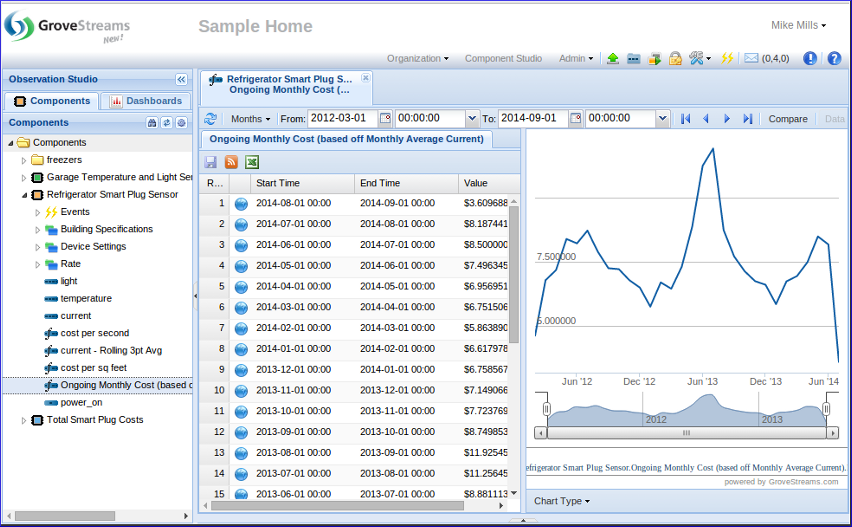Double click on the Ongoing Monthly Cost stream to view ongoing costs. Check for System Job derivation error notifications if the cost stream is not being derived by clicking on the notification button in studio toolbar.

Time of Use
Some utility rates apply a different price based on the day of the week and the time of the day. These are referred to as "Time of Use" rates. To model a Time of Use rate:
1. Select the Tools folder on the left and create a Time Filter for each time of use range by right clicking on the Time Filters folder and selecting New. Some common filters are Summer On Peak, Summer Off Peak, Winter On Peak, Winter Off Peak.
2. Create a new Interval stream for each range, derive it from the current (amperes) stream, and assign the appropriate Time Filter on the Constraints folder. These streams are often referred to as Billing Determinate streams. Each stream represents usage for a specific Time of Use range. The time filter will cause all calculations to ignore "filtered" out intervals.
3. Use the billing determinate streams within the monthly cost derivation calculation. Apply the appropriate price to each stream's kWh.
Hourly Pricing
Some very large utility customers are charged a different price by the hour or possibly by the minute. Create an hourly (or another size) price stream that contains a price for each interval and use it within the cost derivation formula. For hourly pricing, an Hourly cost stream should be calculated instead of a Monthly cost stream. The month's cost is still automatically calculated as a roll-up virtual stream.

Holidays
Utilities typically treat holidays that fall on a weekday as a Sunday. They will apply Off Peak rates to days that are holidays. GroveStreams does not currently support holidays in energy calculations. Adding this enhancement would not be that difficult. Contact us if you need us to support holidays.